Learn

# Pictographs

360 views

 1 Introduction 2 What are Pictographs? 3 Why Pictographs are used? 4 How pictures or images are used in mathematics for Data Handling? 5 Examples 6 Practice Questions 7 Answers 8 Conclusion 9 Frequently Asked Questions (FAQs) 10 External References

29 January 2021

## Brief History

When human civilizations were evolving, humans started writing with pictures!
In Fact, some early human scripts were pictorial in nature. Pictographs too are based on the same principle of conveying information or data in picture form.

Today, pictograms are used in all kinds of environments, from road signs to laundry instructions, educational graphics, and modern technology.

Pictograms were used worldwide from 9,000 BC and became popular around 4,000 years later when they began to develop into logographic writing systems. But the first pictorial signs appeared in 30,000 BC, in the form of cave paintings.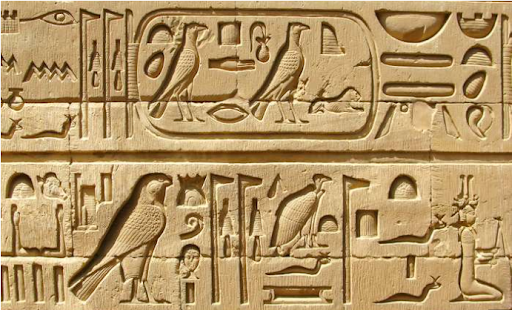## What are Pictographs?

Pictographs are an easy and simple way to enable a reader to understand the data through pictures. Wherein, each image or picture represents a certain amount or number of things.

## Why Pictographs are used?

Pictures and images get easily registered in human memory as they make more impact than the texts. It also conveys information in an easy and very simple way as compared to texts. Images are understandable by anyone who does not even know to read and write.
That is why we also see the usage of images or pictures in a lot of places where an idea or information is conveyed better by the images rather than the texts.

 Examples

(i) Danger signs in high voltage areas.
(ii) Traffic signs
(iii) Images on carton boxes convey that the item is fragile and handle with care.

## How pictures or images are used in mathematics for Data Handling?

Pictographs in mathematics come under the topic of Data Handling.
When we have a lot of data of different types that need to be compared, it is then when we use images or pictures.
So basically an image is chosen which represents a certain number of items. By using this image we can represent any item having any amount of quantity quite easily.
The examples of the same you will find in the example questions.

## How to read and interpret Pictographs?

There are some simple and basic steps that we need to follow in order to understand Pictographs and answer questions based on them.

So let’s understand those steps
Step 1: Identify the image/picture being used to represent data.

Step 2: Figure out how much quantity or what number does that one image represents.

Step 3: Based on the above two observations, solve for what has been asked in the question.

 Examples

Now, let's have a look at some examples to get a better insight into the whole concept of Pictographs!

1. The pictograph shows Priya's collection of different items. Study the graph and answer the following questions.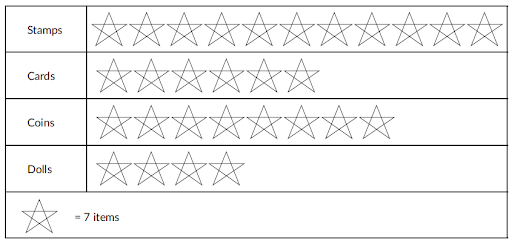How many more cards than dolls does she have?

(A) $$14$$        (B) $$36$$         (C) $$28$$         (D) $$24$$

Solution:

Firstly, we see that the image used to represent data is a Star.
Then we identify that \begin{align}1☆= 7\end{align} items.
So we spot that there are 6☆ against cards and  $$4☆$$ against dolls.
∴ Cards\begin{align}&= {\rm{ }}7 + 7 + 7 + 7 + 7 + 7\,\text{or}\,7 \times 6\\&= {\rm{ }}42\end{align}
Dolls \begin{align} &= {\rm{ }}7 + 7 + 7 + 7\,\text{or}\,7 \times 6\\ &= {\rm{ }}28\end{align}
Cards - Dolls \begin{align}= 42 - 28 = 14\end{align}
∴ Option A

## Practice Questions

Now here are some interesting questions that you can work on based on what we learnt in Pictographs!

1. The pictograph below shows the details of a token exchange counter at a funfair. Children play games, win tokens and exchange them for toys. Study the graph and answer the following questions.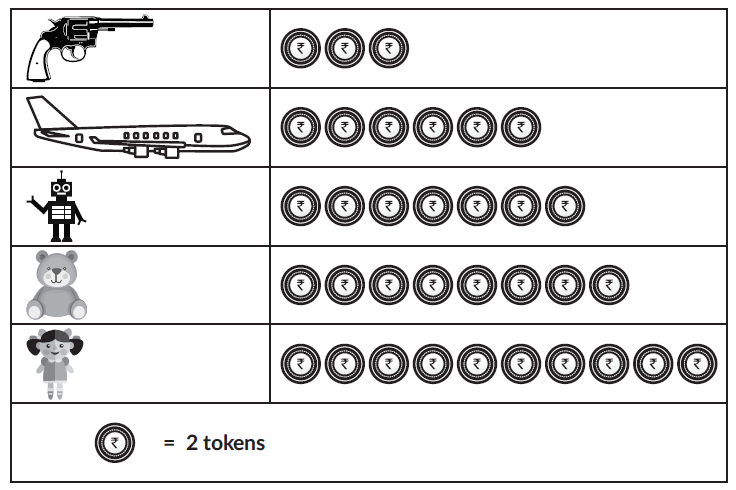Mohit won $$10$$ tokens at the funfair. How many more tokens is needed by him to exchange for a toy robot?

(A) $$3$$         (B) $$1$$         (C) $$4$$        (D) $$2$$

2. How many fewer cheese pizzas than mushroom pizzas are ordered?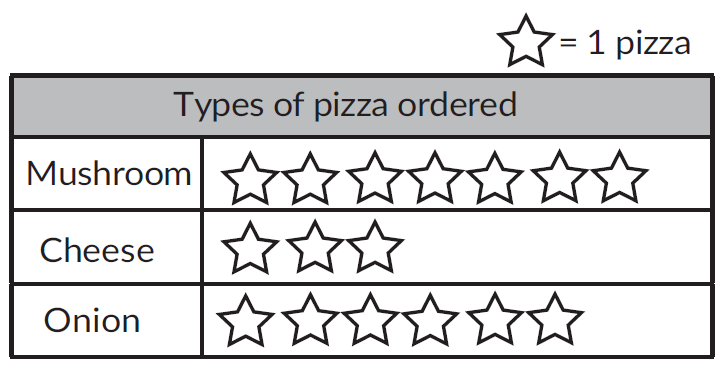(A) $$4$$             (B) $$3$$             (C) $$2$$             (D) $$1$$

3. How many Zebras, Giraffes, and Lions are there in the zoo?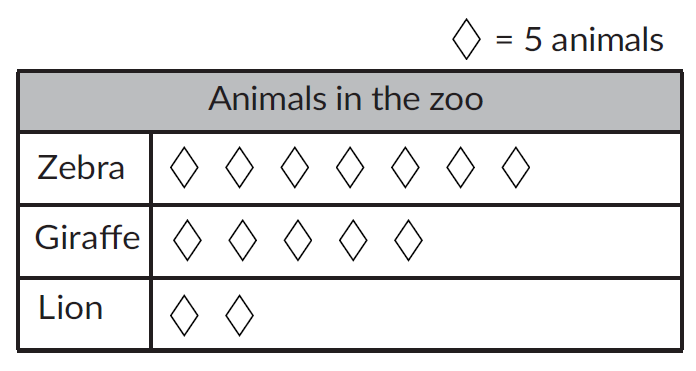(A) $$14$$            (B) $$20$$             (C) $$70$$         (D) $$140$$

4. How many more packs of biscuits than popcorn were sold in the month of January?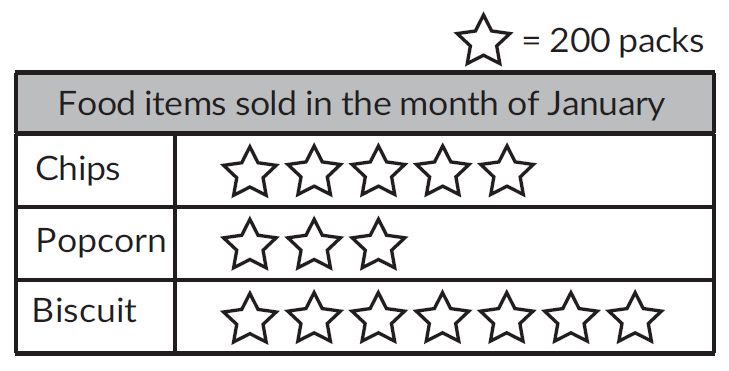(A) $$4$$         (B) $$200$$         (C) $$400$$         (D) $$800$$

 1. Option C 2. Option A 3. Option C 4. Option D

Credits:
: https://www.britannica.com/topic/hieroglyph

## Conclusion

Pictograms are a great addition to an infographic with lots of numbers or text. They are ideal for statistical infographics because they can give a snapshot of quantity and volume in a visually attractive way.

Cuemath, a student-friendly mathematics and coding platform, conducts regular Online Live Classes for academics and skill-development, and their Mental Math App, on both iOS and Android, is a one-stop solution for kids to develop multiple skills. Understand the Cuemath Fee structure and sign up for a free trial.

## How to make a pictograph?

The first step is obviously to collect the data for the category you want to represent. Then choose a symbol or image that accurately represents your data and assign a key. The last step is to draw your pictogram.

## What is a pictograph in maths?

A pictogram is a way of displaying data through images. Each image represents a number of things.

## External References

GIVE YOUR CHILD THE CUEMATH EDGE
Access Personalised Math learning through interactive worksheets, gamified concepts and grade-wise courses